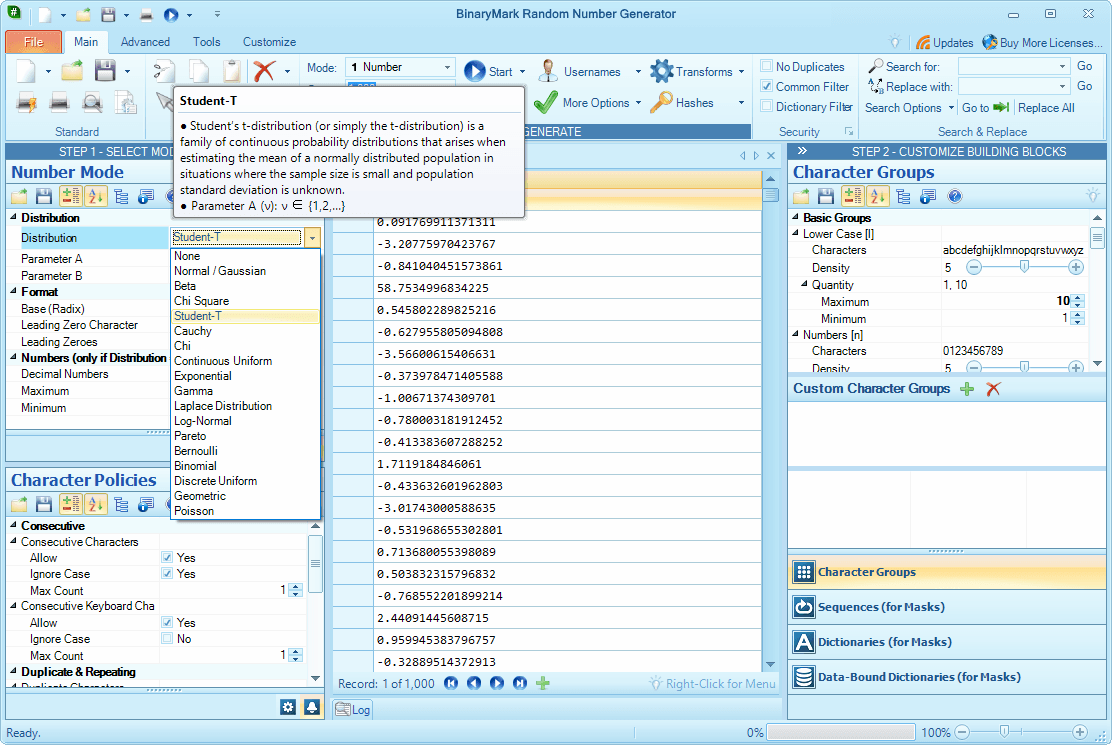# N5 Net Software Repository

Search Files

Only Freeware

### Authors Area

Are you a software author? Take a look at our Author Resource Center where you will find marketing tools, software specifically created for promoting your software and a ton of other helpful resources.

### HIME: HUGE INTEGER MATH AND ENCRYPTION 2.05.1

Development :: Components & LibrariesHIME is a library with encryption functions and huge integer math functions. RSA public key, AES, RC4 secret key encryption, MD5, SHA-512 hash function, random number generation (Blum-Blum-Shub, RSA), Diffie-Hellman, compression, securely erase files. Every programming language that can access a dll can use HIME: C, C++, C#, Visual Basic, VBA, Delphi, PowerBASIC, PureBASIC, Dot NET. The math functions work with huge integers up to 2^31 bits long.
Windows | Shareware

### NUMBERINPUT ACTIVEX 2.0

Development :: Active XThe control element allows the comfortable input of (long) integer values, whereby incorrect inputs by the user become impossible. It is freely adjustable in its size. The size of the represented control sub elements and the size of the displayed numbers adapt automatically. The input of the number can be made directly in the text field or indirectly by the two buttons.
Windows | Shareware

### RANDOM NUMBER GENERATOR 3.0.18

Education :: MathematicsRandomly generate millions of integers or floating point numbers in the desired radix (base), format, and numeric range. Generated random numbers may match any one of the 17 built-in statistical probability distributions: continuous - Normal / Gaussian, Beta, Chi Square, Student-T, Cauchy, Chi, Continuous Uniform, Exponential, Gamma, Laplace, Log-Normal, Pareto; and discrete - Bernoulli, Binomial, Discrete Uniform, Geometric, Poisson.
Windows | SharewareRandomly generate millions of integers or floating point numbers in the desired radix (base), format, and numeric range. Generated random numbers may match any one of the 17 built-in statistical probability distributions: continuous - Normal / Gaussian, Beta, Chi Square, Student-T, Cauchy, Chi, Continuous Uniform, Exponential, Gamma, Laplace, Log-Normal, Pareto; and discrete - Bernoulli, Binomial, Discrete Uniform, Geometric, Poisson.